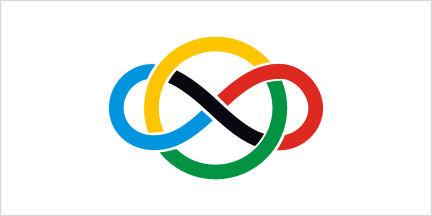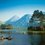# Open Proof Contest 4 - Questions NeededHello Every$\displaystyle \int_{0}^{\pi/2}{\cos{x} dx}$

This is the 4th and the best part of the contest started by me. It's Open Proof Contest. This time the contest will contain 20 questions out of which 10 will be mine and 10 from U all. Don't worry even if U give a question and I put it in the Contest then also u can participate. Those 10 questions will have choices. See each question which will be from you will have a choice abc or xyz. If your question is abc then U have to do xyz and vice versa.

There is no particular topic.U can give any questions except calculus. Give your questions in seperate comments over here. Any doubts feel free to ask. Also don't give the solution to Ur question. until U are asked to. Open till 23th May 11: 59 IST.

Do Reshare

Hoping to see many great questions.:)Note by Rajdeep Dhingra
6 years, 2 months ago

This discussion board is a place to discuss our Daily Challenges and the math and science related to those challenges. Explanations are more than just a solution — they should explain the steps and thinking strategies that you used to obtain the solution. Comments should further the discussion of math and science.

When posting on Brilliant:

• Use the emojis to react to an explanation, whether you're congratulating a job well done , or just really confused .
• Ask specific questions about the challenge or the steps in somebody's explanation. Well-posed questions can add a lot to the discussion, but posting "I don't understand!" doesn't help anyone.
• Try to contribute something new to the discussion, whether it is an extension, generalization or other idea related to the challenge.

MarkdownAppears as
*italics* or _italics_ italics
**bold** or __bold__ bold
- bulleted- list
• bulleted
• list
1. numbered2. list
1. numbered
2. list
Note: you must add a full line of space before and after lists for them to show up correctly
paragraph 1paragraph 2

paragraph 1

paragraph 2

[example link](https://brilliant.org)example link
> This is a quote
This is a quote
    # I indented these lines
# 4 spaces, and now they show
# up as a code block.

print "hello world"
# I indented these lines
# 4 spaces, and now they show
# up as a code block.

print "hello world"
MathAppears as
Remember to wrap math in $$ ... $$ or $ ... $ to ensure proper formatting.
2 \times 3 $2 \times 3$
2^{34} $2^{34}$
a_{i-1} $a_{i-1}$
\frac{2}{3} $\frac{2}{3}$
\sqrt{2} $\sqrt{2}$
\sum_{i=1}^3 $\sum_{i=1}^3$
\sin \theta $\sin \theta$
\boxed{123} $\boxed{123}$

Sort by:

A intermediate problem by me ,

Q 1) Find all '$a$' such that $24\ | \ a^2+34a+1919$

- 6 years, 2 months ago

I think u mean that to be an $a^2$

- 6 years, 2 months ago

yeah... sorry.(typo)

- 6 years, 2 months ago

1) Prove that

$\displaystyle\int_0^{\infty} \ dx \ln^2 \tanh (x) =\dfrac {7}{4}\zeta {(3) }$

$\tanh(x)$ is the hypebolic trigonometry function

You will be provided the solution if you want it. Cheers!

- 6 years, 2 months ago

Nice question. But I already mentioned no Calculus.

- 6 years, 2 months ago

- 6 years, 2 months ago

A intermediate problem by me,

Q1) On a chess-board if two squares are chosen, what is the probability that they have side in common .

- 6 years, 2 months ago

Nice question. But this is a proving contest

- 6 years, 2 months ago

Ok here is my question

prove that $\large{\frac{a^{8}+b^{8}+c^{8}}{a^{3}b^{3}c^{3}}>\frac{1}{a}+\frac{1}{b}+\frac{1}{c}}$ where $a,b,c>0$

- 6 years, 2 months ago

Great! This one surely will make it.

Note: Type new questions in new comments

- 6 years, 2 months ago

Ok should i remove it and type in new comment

- 6 years, 2 months ago

Just reword it to "prove that the probability is __".

I guess that makes it a proof :3

- 6 years, 2 months ago

Maybe. But I will see to it.

- 6 years, 2 months ago

You want algebra problem?

Let $a,b,c$ be positive reals satisfying $a^3+b^3+c^3+abc=4$ . Prove that $\frac{(5a^2+bc)^2}{(a+b)(a+c)} + \frac{(5b^2+ca)^2}{(b+c)(b+a)} + \frac{(5c^2+ab)^2}{(c+a)(c+b)} \ge \frac{(a^3+b^3+c^3+6)^2}{a+b+c}$ .

###### BONUS : How many cases of equality are there?

- 6 years, 2 months ago

@Rajdeep Dhingra , check out this algebra question.

- 6 years, 2 months ago

A slightly-above-intermediate problem by me.

Q #1 .

$\quad$

Find all integral solutions to the following equation:

$\quad$

$\frac {1}{m} + \frac{1}{n} - \frac {1} { mn^2} = \frac {3}{4}$

- 6 years, 2 months ago

Nice question ! Thanks.

- 6 years, 2 months ago

An easy proof problem :

Prove that $n!$ > $2^{n}$ for all integers $n \geq 4$.

- 6 years, 2 months ago

Just out of curiosity , what level would you classify this question in?

- 6 years, 2 months ago

Level 1 or 2 maybe. What about you?What lvl will you classify this?

- 6 years, 2 months ago

Level 2-3.IMO , the method of solving this problem is not so trivial to be classified as a level 1 or 2.(I'm trying not to give away the solving method I think one may "INDUCT" electricity otherwise?! xD)

- 6 years, 2 months ago

Lollololl :P

- 6 years, 2 months ago

This is a nice question but not original :

Q #2) $n$ circles are drawn with a radii length of 1 unit where $n \ge 2$ , such that every two circles intersect.Prove that the number of intersection points is at least $n$.

- 6 years, 2 months ago

Lemme post a geometry problem :

In $\Delta ABC$ , if $BC^2=AC(AC+AB)$ , then prove that $m\angle CAB = 2 \times m\angle CBA$.

- 6 years, 2 months ago

Respected sirs,

- 6 years, 2 months ago

@Archit Boobna @Parth Bhardwaj see this

- 6 years, 2 months ago

I think you meant 24th May in the deadline. I have corrected it accordingly.

- 6 years, 2 months ago